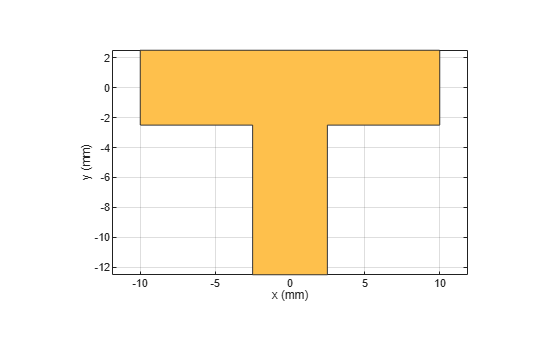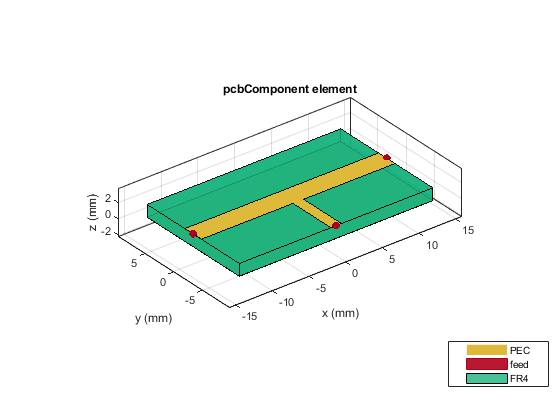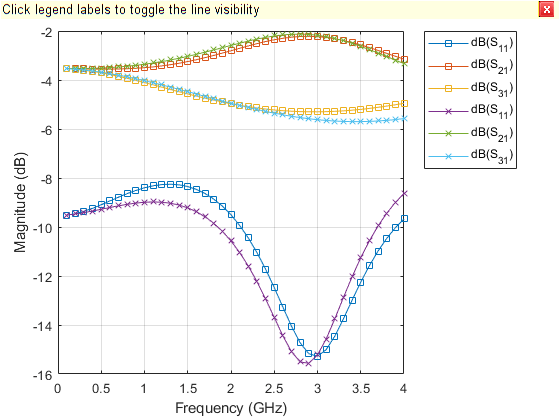traceTee

Create tee trace

Description

Use the traceTee object to create a tee trace on the X-Y plane.

Creation

Description

example

trace = traceTee creates a tee trace with default properties on the X-Y plane.

trace = traceTee(Name=Value) sets Properties using one or more name-value arguments. For example, traceTee(ReferencePoint=[1 1]) creates a tee trace with the reference point [1 1]. Properties not specified retain their default values.

Properties

expand all

Name of the tee trace, specified as a character vector or a string scalar.

Example: trace = traceTee(Name="traceTeeShape")

Data Types: char | string

Reference point of the tee trace in meters, specified as a two-element vector of nonnegative elements.

Example: trace = traceTee(ReferencePoint=[1 1])

Data Types: double

Length of the horizontal and vertical lines in meters, specified as a two-element vector of positive elements.

Example: trace = traceTee(Length=[0.0300 0.0200])

Data Types: double

Width of the horizontal and vertical lines in meters, specified as a two-element vector of positive elements.

Example: trace = traceTee(Width=[0.0060 0.0060])

Data Types: double

Offset along the X-axis in meters, specified as a nonnegative scalar.

Example: trace = traceTee(Offset=0.0005)

Data Types: double

Object Functions

 add Boolean unite operation on two RF PCB shapes subtract Boolean subtraction operation on two RF PCB shapes intersect Boolean intersection operation on two RF PCB shapes plus Shape1 + Shape2 for RF PCB shapes minus Shape1 - Shape2 for RF PCB shapes and Shape1 & Shape2 for RF PCB shapes area Calculate area of RF PCB shape in square meters rotate Rotate RF PCB shape about defined axis rotateX Rotate RF PCB shape about x-axis rotateY Rotate RF PCB shape about y-axis and angle rotateZ Rotate RF PCB shape about z-axis translate Move RF PCB shape to new location scale Change size of RF PCB shape by fixed amount

Examples

collapse all

Create a tee trace with default properties.

trace = traceTee
trace =
traceTee with properties:

Name: 'mytraceTeeShape'
ReferencePoint: [0 0]
Length: [0.0200 0.0100]
Width: [0.0050 0.0050]
Offset: 0

View the trace.

show(trace)Design a microstrip transmission line at 3 GHz for FR4 substrate.

m = design(microstripLine('Substrate',dielectric('FR4')),3e9);

Create a microstrip T-junction.

layer2d = traceTee('Length',[m.Length m.Length/4],...
"Width",[m.Width m.Width/2]);

Convert the T-junction trace to a 3-D component.

robj = pcbComponent(layer2d);
robj.BoardThickness = m.Substrate.Thickness;
robj.Layers{2} = m.Substrate;
show(robj)Define frequency points to calculate the s-parameters.

freq = (1:40)*100e6;

Calculate the s-parameters of the T-junction trace using the behavioral model.

Sckt = sparameters(robj,freq,75,'Behavioral',true);
Warning: Behavioral model is valid only when Z0 of main line is 50 ohms and for EpsilonR of 9.9.

Calculate the s-parameters of the T-junction trace using the electromagnetic solver.

Sem = sparameters(robj,freq,75)
Sem =
sparameters: S-parameters object

NumPorts: 3
Frequencies: [40x1 double]
Parameters: [3x3x40 double]
Impedance: 75

rfparam(obj,i,j) returns S-parameter Sij

Plot the s-parameter data using the rfplot function.

rfplot(Sckt,1,1,'db','-s')
hold on
rfplot(Sem,1,1,'db','-x')
rfplot(Sckt,2,1,'db','-s')
rfplot(Sem,2,1,'db','-x')
rfplot(Sckt,3,1,'db','-s')
rfplot(Sem,3,1,'db','-x')References:

1. Ramesh Garg & I. J. Bahl (1978) Microstrip discontinuities, International Journal of Electronics, 45:1, 81-87, DOI: 10.1080/00207217808900883

2. Wadell, Brian C. Transmission Line Design Handbook. The Artech House Microwave Library. Boston: Artech House, 1991.